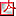from DSSResources.com

## What is a decision support model?

by Dan Power
Editor, DSSResources.com

In the realm of decision support, a model is a quantitative description of a phenomenon. A model replicates major properties of a phenomenon of interest and can be used to study it. A model is an abstraction of a real system or thing. In general, a quantitative model attempts to link the major variables or elements of a system in such a way that results can be generated that are predictive of the behavior of the actual system.

In broad terms, a quantitative description means that inputs and outputs are processed by the variables that describe any transformations that occur as part of a phenomenon. The model has a boundary and both inputs and outputs cross the boundary. The values of inputs are processed by the model to determine the values of outputs. A simple example may help explain the decision support model. Let's describe a cost estimation model where total cost is equal to number of labor hours multiplied by the wage rate per hour. So TC = N * \$12.00, where \$12.00 is the wage rate. N is the input and TC is the output. The model describes the transformation of the input to determine the output. If N = 30, then TC = \$360.00. This example is a simple algebraic model.

Last update: 2013-01-13 06:26
Author: Daniel PowerPrint this recordShow this as PDF file

Average rating: 5 from 5 (1 Votes )

completely useless 1 2 3 4 5 most valuable

You cannot comment on this entry

 DSS Home |  About Us |  Contact Us |  Site Index |  Subscribe | What's NewCopyright © 1995-2015 by D. J. Power (see his home page). DSSResources.COMsm is maintained by Daniel J. Power. Please contact him at djpower1950@gmail.com with questions. See disclaimer and privacy statement.Web DSSResources.com